# Machine Learning Glossary

Alex Egg,

## TODO

• D trees (probably code one to learn it best):
• Pretty much did this for classification of numerical and categorical variables. **Didn’t explore regressions though. (This bit me w/ BCG :( ) **.. Think they just take the mean of the leaf values
• Simple GAN w/ mnist (port my TF example to Keras)
• Boosting
• One cycle FastAI stuff for LR scheduling
• Seq2Seq Attention
• Quantile Regression
• Revisit SVD in context of Rec Sys and Dimensionality Reduction (dim reduction vs PCA)
• Revisit SVMs
• Maximum likelihood questions: solve exponential function and get the maximum likelihood estimator.
• Probability questions: There are 100 product and 25 of them is bad. What is the confident interval.
• LDA connection to PCA
• MLE ME MAP <- learn these, especially MLE
• Probability Calibration (scikit)
• The distribution of predictions should match the distribution of of the data. If it is skewed, it should be corrected (calibrated). This could also be connected to Netflix’s Calibrated Recommendations at recsys ‘18.
• Simplex Algorithm (liner programming) <-asked if you can solve a linear equation
• ordinary least squares vs partial least squares
• Survivorship analysis (long tail processes re Better Mortgage)
• Generative Models
• He asked if I work w/ them and I must admit I don’t think like that. VAEs… GANs…
• Input Normalization: standard procedure for gradient optimization. BUT is it required for Least Squares???
• If you have a FC net 5 layers deep and you go to 7 and see no gains. Why?

## Information Theory

### Shannon Entropy

Information entropy is the average rate at which information is produced by a stochastic source of data.
$\text{Entropy} =- \sum_i p_i\log_2p_i$
Where $p_i$ is the probability of class $i$. In the binary classification case, we can rollout the summation to:
$\text{Entropy}= -p\log_2p-q\log_sq$
Where p and q are the respective categories.

• What is the entropy of a homogenous set?
• $-1 \log_21 - 0\log_20 = 0$
• What is the entropy of a set w/ a 50/50 mix?
• $(-.5\log_2.5) - (.5\log_2.5) = 1$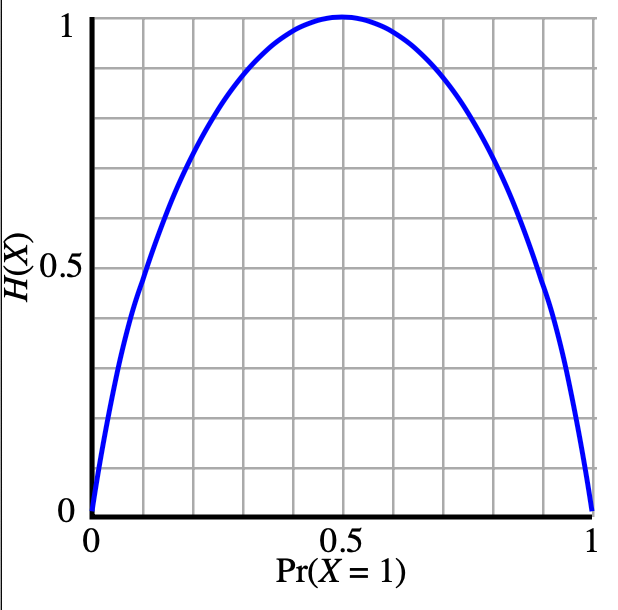Figure: Distribution of entropy for binary random variable (coin). For a fair coin p=.5, there is maximum entropy.

• DFS: Traverse down branches in lexical order
• Hill: Traverse down branches in using heuristic. Heuristic is shortest path to goal.
• BFS: Exhaustively traverse across branches
• Beam: Heuristic to traverse only best $k$ branches

Recursive DFS tree traversal

def traverse(node, visited):
visited.add(node)

for node in node.children - visited:
traverse(node, visited)

if len(node.children)==0:
#leaf
return

traverse(root, set())


Recursive BFS tree traversal:

q = []
def traverse(node, visited):
while q:
node = q.pop()
visited.add(node)

for node in node.children - visited:
queue.append(node)

traverse(root, set())


## Linear Algebra

### Norm

Measurement of the magnitude of a vector. Typically used for quantifying model parameter complexity, where complexity is viewed as either vector magnitude or sparseness.

• L0
• L1 (Manhattan): $\norm{x}1 = \sum{i=0} \abs{x_i}$
• L2 (Euclidian): $\norm{x}2 = \sqrt{\sum{i=0}x_i^2} = \sqrt{x\cdot x…}$
• Frobenius: Matrix Norm

### Distance

Distance measure between two vectors $A$ and $B$

• Euclidian: $norm(A-B)$ which is to say the size of the difference of the vectors
• Dot Product : $a\cdot b = \norm{A}\norm{B} cos(\theta)$ (loose measure of similarity. Bigger if a and b are more simular)
• Cosine: Normalized dot product : $\theta = 1- \frac{a\cdot b}{\norm{A}\norm{B}}$ (you can see the dot product in numerator, but then it’s divided by the lengths of the vectors in denominator)

Dot Product = $\sum_i \sum_j i\cdot j$

Cosine: normalized dot product (angle between two vectors)

### Computations

Dot product is a $O(n)$ scalar operation. Can a vectorized operation run faster? BLAS etc… Is it faster in just wall time or is it less complex?

Same study for Matrix Multiply $O(n^3)$

## Statistics

• Central Limit Theorem: Consider the sum of multiple random variables. The sum of a set of random variables, which is of course itself a random variable, has a distribution that become increasingly Gaussian as the number of terms in the sum increases.
• I think this is useful in hypothesis testing if you run a bunch of random experiments, you can assume the distribution of the results is Normal/Gaussian (even if the individual random variable isn’t normally distributed) and then do a t-test.
• Do the hypothesis testing exercise on data quest which I always thought was nice!
• Variance: how one variable varies with itself: $\frac{1}{n}\sum_{i=0}^x (x_i - \hat{x})^2$ (sum of squared diffs from mean)
• Covariance: how one variable varies w/ others: $\Sigma$ (mean of the product of each variables variance). If $X$ and $Y$ are independent, then $Cov(X,Y)=0$
• Correlation: Measure of the linear relationship between two variables. Takes value between +1 and −1 inclusive: $Corr(X,Y) = \frac{Cov(X,Y)}{\sqrt{Var(X)Var(Y)}}$ (normalized variance)

## Probabilities

• Conjunctive: probability of Event1 AND event2; multiply probabilities
• Disjunctive: probability of Event1 OR event2; add probabilities
• Conditional: probability of A given B $P(A\given B)$ : $\frac{P(A \cap B)}{P(B)}$
• Bayes Rule: $P(A\given B) = \frac{P(B \given A) P (A)}{P(B)}$

### Bayes Rule

Example: There is a cancer test that is 75% accurate (TP Rate/sensitivity):
$P(X=1|Y=1) = .75$
The prior probably of having cancer in general is $P(Y=1)=.01$

The probability of a positive test $P(X=1)$:
$P(X=1) = P(X=1|Y=1)P(Y=1) + P(X=1 | Y=0)P(Y=0) =\\ .75*.01 + .25*.99= 0.255$
What is the posterior probably $P(Y\given X)$ that you have cancer if you have a positive on the test?
$P(Y|X) = \frac{P(X=1|Y=1) P(Y=1)}{P(X)} = \\ \frac{P(X=1|Y=1) P(Y=1)}{P(X=1|Y=1) P(Y=1) + P(X=1|Y=0) P(Y=0)}= \frac{.75 * .01}{.255} =.029$

### Expectation Notation

For an unfair or weighted coin, the two outcomes are not equally likely. You can change the weight or distribution of the coin by dragging the true probability bars (on the right in blue) up or down. If we assign numbers to the outcomes — say, 1 for heads, 0 for tails — then we have created the mathematical object known as a random variable.

The expectation of a random variable is a number that attempts to capture the center of that random variable’s distribution. It can be interpreted as the long-run average of many independent samples from the given distribution. More precisely, it is defined as the probability-weighted sum of all possible values in the random variable’s support.
$E[X]=\sum_{x\in x}xP(x)$
Which equals 3.5 for a dice: $1/6 + 21/6… +61/6$

Variance: Whereas expectation provides a measure of centrality, the variance of a random variable quantifies the spread of that random variable’s distribution. The variance is the average value of the squared difference between the random variable and its expectation,
$Var(X) = E[(X-E[X])^2]$

## Distributions

• Skew: measures clustering of distribution. If you have a right skew it’s common to normalize the data (log).
• Kurtosis: measures the shape of distribution
• Modality: measures tendency of distribution
• Mean: measures central tendency but is sensitive to outliers (skew)
• Median: measures central tendency and is less sensitive to outliers (skew)
• Mode: most common number in dist
• Variance, $σ^2$: measures how far the average data point is from the mean: $\sum_i\frac{(\mu - x_i)^2}{\mu}$
• Standard deviation, σ: common way to refer to the distance between data points and the mean.
• Stationary: Mean and variance are constant. Typically seen In time-series data.
• Normalization: Makes the data stationary meaning for random windows the mean and variance are constant
• Bernoulli Trail: Fail or success
• KL Divergence: Test similarity of two probability distributions. Minimizing the KL loss is generally used in MLE.
• Confidence Interval

• Normal: We can generate a normal distribution by using a probability density function.

• Parameterized by $\mu$ and $\sigma$
•• Binomial: sequential binary action: rain/dry, basketball shot/miss, market up/down

• Parameterized by: $n$ and $p$ number of events and prob of success respectively
•• Unimodal: One mode (normal)

• Multimodal: More than 1 mode• Poisson: Number of calls received per hour by call center (when you want a normal w/o negative values…)

• Parameterized by $\lambda$• Exponential: a skewed distribution that can model user time on a page in seconds

• Parameterized by $\lambda$### Questions

• What is the expected value of flips to get heads on a fair coin?
• $E(x) \sim \text{Geometric}$, Expected value = $\frac{1}{p}$ = , i.e. is 1/.5 = 2
• Given a random Bernoulli trial generator, write a function to return a value sampled from a normal distribution.
• The trick is to sample from the normal CDF which samples from the Normal PDF

## Experiment Design

• Null Hypothesis: Status Quo, random chance
• Alternative Hypothesis: statistically significant difference between the outcomes of the 2 groups.
• One-sided: null < x, alt >=x; Two-sided (true/false): null=0, alt!=0
• Blind Experimentation:
• Control Group & Treatment Group
• Test Statistic: quantifies the data in the groups
• Statistical Test: Use Test Statistic to ran experiments
• P-value: probability quantifying random chance. The lower the better.
• Sample Size: refers to how large the sample for your experiment is. The larger your sample size, the more confident you can be in the result of the experiment (assuming that it is a randomized sample).
• $n = 16 \frac{\sigma^2}{\delta^2}$
• Effect Size/Minimum Detectible Effect $\delta$: The Minimum Detectable Effect is the smallest effect that will be detected $power\%$ of the time.
• refers to the size of the difference in results between the two sample sets and indicates practical significance. If there is a small effect size (say a 0.1% increase in conversion rate) you will need a very large sample size to determine whether that difference is significant or just due to chance. However, if you observe a very large effect on your numbers, you will be able to validate it with a smaller sample size to a higher degree of confidence. %5 is a common number.
• Confidence Interval: product of sample size and effect size. $\mu +-Z\frac{\sigma}{\sqrt(n)}$
• Chai-Squared: Does the rate of success differ across two groups?
• Significance: Statistical significance is the likelihood that the difference in conversion rates between a given variation and the baseline is not due to random chance. ie if you run an A/B testing experiment with a significance level of 95%, this means that if you determine a winner, you can be 95% confident that the observed results are real and not an error caused by randomness.
• Significance Level $\alpha$: usually 0.05
• Type I error/Confidence Level (1-⍺) — Chance of accepting a difference when there is not. 90%, 95%, 99%(a=.01)
• Type2 error $\beta$ - Chance of rejecting an actual lift. β is inversely related to sample size.
• Statistical Power  — (1-β) is the power or sensitivity of experiment
• Product of Sample Size and Effect Size $\delta$
• Causal Inference???
• Confounding Factors: Causation is not correlation. unknown variables that might be influencing the test. For example, my conclusion is that Shoe Size is correlated w/ Literacy. However, the confounding factor there is age, which is actually correlated w/ literacy.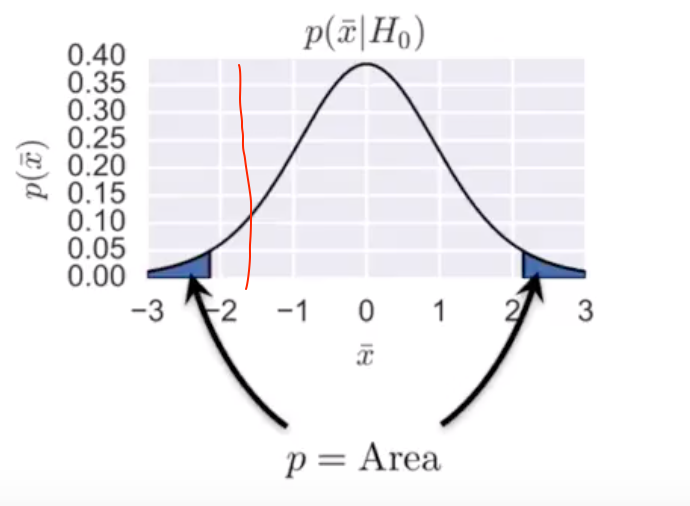Figure: A normal/t distribution for the null hypothesis where $\mu=0$ w/ 2 tails highlighted being the regions the sample $\mu$ must lie for the p value to be below 0.05. You can see the sample mean lies between, -2 and -1 and the goal of the test is to see if this is “weird” or not. It is weird if it is in the tail.

### Statistical Tests

• Covariance: relationship between the variances of two variables

• Correlation: two variables change together

• Pearson: r-value (continuous variables)
• Spearman: ordinal variables
• $\chi^2$ Test: Similarity of two discrete categorical distributions (usually frequency distributions)

• Comparison of Means

• T-test: Tests for the difference between two related variables (normality assumption) when you don’t know the population variance. (Otherwise z-test)

• scipy.stats.ttest_ind https://docs.scipy.org/doc/scipy-0.15.1/reference/generated/scipy.stats.ttest_ind.html#scipy-stats-ttest-ind

• #test w/ idential means

>>> rvs1 = stats.norm.rvs(loc=5,scale=10,size=500)
>>> rvs2 = stats.norm.rvs(loc=5,scale=10,size=500)
>>> stats.ttest_ind(rvs1,rvs2)
(0.26833823296239279, 0.78849443369564776) #accept null

#T-test with different means, variance, and n:

>>> rvs5 = stats.norm.rvs(loc=8, scale=20, size=100)
>>> stats.ttest_ind(rvs1, rvs5)
(-1.4679669854490653, 0.14263895620529152) #reject null

• Welschs t-test: (improvement over classical t-tests) comparison of two means (assumes normality)

• Z-test: when we know the population $u$ and $\sigma$

### Questions

• In the experiment design portion you are asked to create a hypothetical plan to answer testing a new app.

• Identify Goals
• Identify Invariant and evaluation metrics
• Create Hypothesis
• Choose the test statistic
• Choose the statistical test (two sample tests): t-tests, $X^2$ test, etc
• Choose significance level ($\alpha$), statistical power (1-$\beta$) and Effect Size $\delta$
• Significance Level is usually $\alpha=0.05$
• Power: How many events do we need in test/control to get a statistically significant result. Usually $1-\beta = 0.8$
• Choose Unit of Diversion & Sample size
• How will you split the users among tests? Page view, cookie or user id? Also consider what population will get a test, how to segment your users?
• Can you accurately identify unique users across devices, sessions etc. What if a users changes devices, can you still uniquely identify them. This is important for splitting!.
• One technique that came up in my call w/ Wayfair was that you can build device finger prints, or features for each device, build these pairs and link them w/ the logged in user as a boolean label. Then learn a log reg that can classify unobserved pairs. What you are interested in is the probabilities. Then you can sorta build a probabilistic graph w/ two nodes, users and devices and edges are probities they are same user.
• Run Experiment
• Analyze results for significance
• Check invariants
• Check for significance in result
• explain p-value

• Probability that the null hypothesis is true. Typical cutoff is .05 5% so anything under that we reject the null hypothesis, meaning that the effect is not due to randomness.
• In an AB test, how to measure regression?

• If the test statistic is lower than the control baseline

### Feedback

• To decide which of two models is better, proposed an experiment design where some metrics (like latency) stay invariant and some change (like CTR)
• Explained the intuition between confidence bounds and number of impressions (the more impressions, the more confident we are)
• Could not describe any statistical test or how to estimate confidence bounds
• Get sample mean.
• Get Z score from table (90, 95 or 99%)
• Get standard error = $\frac{\sigma}{\sqrt(n)}$

## Estimation

### MLE Maximum Likelihood Estimation

Method to fit data to a distribution. Goodfellow (2016) gushes over it.

When you try MLE on a gaussian you get MSE. When you try MLE on a Binomial you get Binary X-Entropy. This means that implicitly when you use MSE, you are doing regression (continuous estimation) and when you use X-Ent, you are doing (binary) classification.

MLE is a special case of Maximum A-posteriori Estimation (MAP) where the prior is uniform.

#### Normal

Given some measurements (ie weight of rabbits), get the normal distribution likelihood function which is parameterized by $\mu$ and $\sigma$ and then maximize the likelihood function to find the estimates for the parameters that fit the data.

MLE works down to:

****DERIVE MSE FROM NORMAL MLE**
$\sum (y-\hat{y})^2$

#### Binomial

Given some bernuli samples (ie free throws ) try to fit the binomial distribution, which is paramteramized by $p$, to the data. Get the PMF of the Bernoulli Distribution:
$f(x_i \given p) = p^x (1-p)^{1-x}$
The Likelihood function $L(p\given x)$ is the product of each data point given the parameter:
$L(p|x_i, ... x_n) = \prod^n_{i=1} f(x_i|p) = p^{\sum^n_{I=1}x_i}-(1-p)^{\sum^n_{I=1}1-x_i}$
Take the log for connivence down the road:
$(\sum^n_{i=1} x_i) \log p + \sum^n_{I=1}(1-x_i) * \log (1-p)$
And you have recovered binary cross entropy ^^^^

Take derivative w/ respect to $p$ and solve for 0.

#### Poisson

Random sample of the number of textbooks that a person buys for a semester at uni.

Poisson Dist is parameterized by $\lambda$ . PDF:
$f(x_i | \lambda) = \frac{e^{-\lambda} \lambda^{x_i}}{x_i!}$
Now compose Likelihood function:
$L(\lambda | x_i...x_n) = \prod^n_{i=1} f(x_i | \lambda)$
Log and take derivative at 0 to get the MLE estimator of $\hat\lambda_{MLE} =\frac{ \sum x_i}{ n}$

### MAP Estimation

MLE is a special case of maximum a posteriori estimation (MAP) that assumes a uniform prior distribution of the parameters.

Includes Prior knowledge!

Bayes Rule:
$P(\Theta | D) = \frac{P(D|\Theta) P(\Theta)}{P(D)}$
Where $P(\Theta)$ can be a uniform distribution and thus ignored and $P(D)$ is considered a constant and thus ignored.

Or equivalently:
$P(\Theta|D) \propto P(D|\Theta)P(\Theta)$
Where the first part is the posterior and the second part is the likelihood prior.

• When is MAP same as MLE?
• When there is no prior or when the prior is uniform.

## Expectation Maximization

Lambda Loss Paper uses this….

A way to calculate MLE/MAP

EM is usually useful when it’s hard to calculate the gradient of the log-likelihood. In cases I’m familiar with, this is due to the presence of latent variables per datapoint. You see it in Gaussian mixture models, hidden Markov models, and other problems. In order to avoid calculating the gradient, you iteratively:

• take the expected complete data log-likelihood
• maximize it

This is guaranteed to converge to a local optimum.

## Time Series Analysis

Classical TS modeling is Generative: one tried to model the process that generates the signal. The new package Prophet, is a Discriminative model which tries to fit a line to the data.

• Auto Correlation: Univariate analysis (denoted by an ACF plot) which shows the correlation between a variable and it’s lag (some period prior). This state violates the Statistical independence of observations assumption of Linear Regression, so when this condition exists and may call for more advanced modeling than simple Linear Regression (see AR Modeling).
• Running correlation analysis on the same variable (not two). So $(y_i, y_{i-1}), (y_{I-1}, y_{I-2})$
• How strongly are todays temperatures related to yesterdays?
• Auto Regressive: a term used to describe the process/model (aka Autoregressive model) when one value may be affected by prior values. Current value is based on the immediately preceding value. Therefore an AR model assumes some degree of auto correlation. Like Linear regression assumptions require the absense of AC. An AR model doesn’t have traditional features. It has auto-correlated features. The feature are lagged responses (not predictors).
• AR models, meaning regression models that use the past as input features, are no different from regression with time-unrelated features. What we are doing is just using previous y-values as input for a given point. We can manually construct such features directly.
• When we add a 2-lag feature (meaning grosses from two weeks ago & last week in our case), the model is called AR-2 (AR for AutoRegressive). When we use up to three, it is called AR-3 and so on. Let’s do an AR-2.

### Autocorrelation Function Plot

The autocorrelation function is a measure of the correlation between observations of a time series that are separated by k time units (yt and yt–k).

Use the autocorrelation function and the partial autocorrelation functions together to identify ARIMA models. Examine the spikes at each lag to determine whether they are significant. A significant spike will extend beyond the significance limits, which indicates that the correlation for that lag doesn’t equal zero.

The data should be stationary before you interpret the autocorrelation plot. A stationary time series has a mean, variance, and autocorrelation function that are essentially constant through time.ARIMA: Auto Regressive Integrated Moving Average

Dilated Causal Conv Net

• GMM
• VAE
• GAN

## Discriminative Models

Everything Else!

• Logistic regression
• Support Vector Machines
• Maximum-entropy Markov models
• Conditional random fields
• Neural networks

## ML

• Auto Correlation: where outputs depend on past inputs.

### Class Imbalance

Good to look at recall

• Down/Up Sample: balance class weights, however, you throw away a lot of data
• Weighted Loss: Weight the loss function by the inverse class frequency count
• SKLearn has a class_weights param which scales the loss.
• Robust Modeling: Use a model that isn’t sensitive to class imbalance:
• Treat as anomaly detection problem: Isolation Forrest
• Boosting: sequential models will adapt to imbalance

### Feature Engineering

#### BOW (Vocabularies)

When you have a variable list of features (like words in a sentence, or number of products a user liked), then a common pattern to is transform this into a fixed length feature vector across the whole vocabulary $V$.

#### Buckets (Continuous Features)

Continuous features don’t need extra treatment, however, signal can be gained by knowing their properties. These tricks can help you stay on a linear model :)

Looking at the distribution of features, we can define relevant buckets for continuous variables, such as counters or timestamps. For instance, consider the number of products a user has seen. Seeing four products definitely reflects more engagement than seeing only one product. However, seeing a hundred and four products is not that different from seeing a hundred products, hence the need to bucketize continuous variables.

Interesting case study from scikit: https://scikit-learn.org/stable/auto_examples/preprocessing/plot_discretization.html#sphx-glr-auto-examples-preprocessing-plot-discretization-py

In 1D the linear regression can’t fit the data, but in 10 dimensions it can! This is because the inflexible linear model can’t fit the non-linear curve in 1d. However, if we let it fit in multiple dimensions, we can gain some expressiveness.

For example, consider raw data that represents the year a house was built. Instead of representing that year as a scalar numeric column, we could split the year into the following four buckets: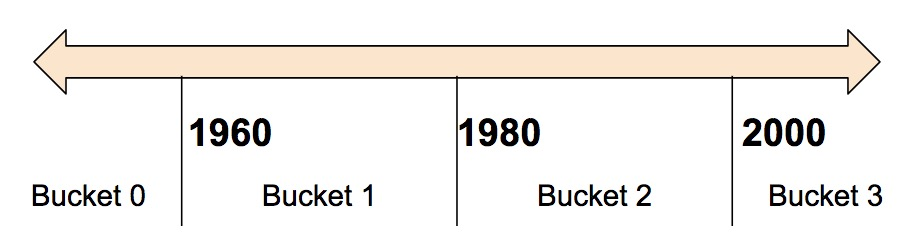Date Range Represented as…
< 1960 [1, 0, 0, 0]
>= 1960 but < 1980 [0, 1, 0, 0]
>= 1980 but < 2000 [0, 0, 1, 0]
>= 2000 [0, 0, 0, 1]

Why would you want to split a number—a perfectly valid input to your model—into a categorical value? Well, notice that the categorization splits a single input number into a four-element vector. Therefore, the model now can learn four individual weights rather than just one; four weights creates a richer model than one weight. More importantly, bucketizing enables the model to clearly distinguish between different year categories since only one of the elements is set (1) and the other three elements are cleared (0). For example, when we just use a single number (a year) as input, a linear model can only learn a linear relationship. So, bucketing provides the model with additional flexibility that the model can use to learn.

#### Feature Crosses

Moreover, some variables make more sense when crossing them together to analyze the co-occurrence effect, such as the number of banner a user has seen on a particular device. The “fatigue” effect is heavily dependent on the screen used, and consequently on the device type the user is on. See factorization machines.

##### Latitude Longitude

Latitude and Longitude as numerical features alone don’t give much discriminative power. An interesting technique that I like is doing bucketed feature crosses. So first you discretize by some type of bucketing to to make lat and long ranges, then you do feature crosses on the ranges to make geo squares!

How do you handle cases where a point on the map is on the boundary of one of your grid squares? Reminds me of the time encoding task….

#### Embeddings

Learnt dense representation of sparse (categorical) data.

Anytime you have a categorical feature, you can probably learn an embedding

#### Transformations

Cartesian Space: Time-of-day

Encoding the numerical Time-of-day as categories from 0-23 ruins the continuity from 12 am to 1am. Instead consider time a vector in polar space and then convert to cartesian space. This transformation will give you two dimensions in cartesian space.

The naive way to do this is to bucketize into parts of day or to make make a continuous feature out of the hours. But there is a more sophisticated was using trigonometry.

The problem of using hour of day is that it ruins the circular nature of time by adding a discontinuity between 12PM and 1AM. Instead, consider time as an angle w/ a unit vector and then covert that to 2d cartesian space.

The discontinuity is bad b/c we are saying there is a relative importance between 2am-12pm and not 12pm-1am when there really is!

Technically, this means adding two new features x,y in cartesian space. Now hour 23 and 0 are right next to each other.

import numpy as np

df['hr_sin'] = np.sin(df.hr*(2.*np.pi/24))
df['hr_cos'] = np.cos(df.hr*(2.*np.pi/24))
df['mnth_sin'] = np.sin((df.mnth-1)*(2.*np.pi/12))
df['mnth_cos'] = np.cos((df.mnth-1)*(2.*np.pi/12))


### Dimensionality Reduction

• Feature Selection
• SVD
• PCA
• Random Projections

### KMeans Clustering

Clustering method which tries to minimize the in-cluster SSE. Parameterized by $K$ for the number of clusters/centroids. Very sensitive to the initial location of the centroids.

1. Initiation of centroids (random or heuristic – very important)
2. Assignment of data points to 1NN centroids
3. Update centroids location (center of cluster/mean of cluster points)
4. Repeat #2 and terminate upon convergence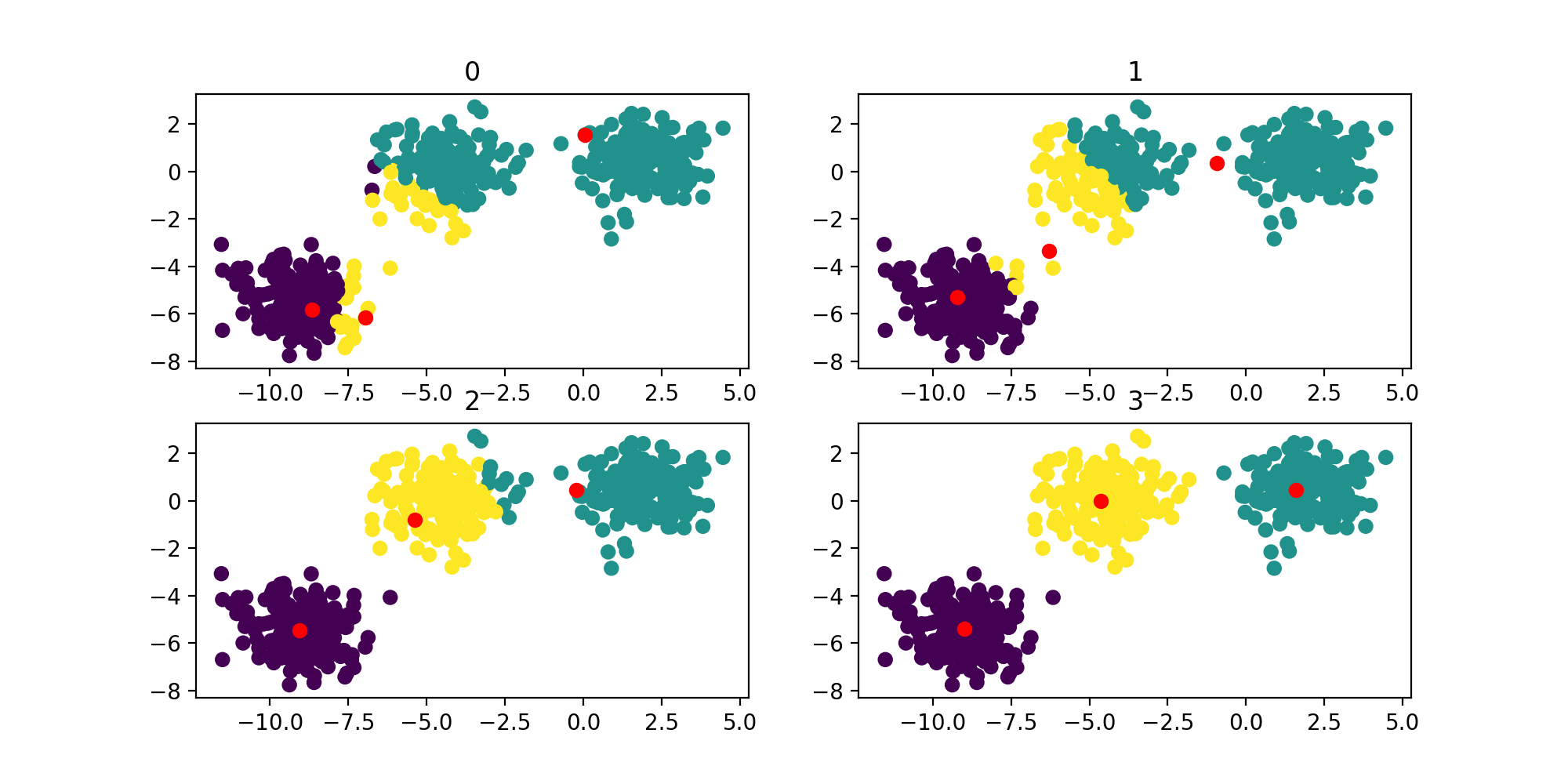Figure: KMeans run for 4 iterations

### Linear Regression

R2
$R^2 = 1-\frac{SSE}{SST}$
Where SEE is the mean squared loss of the optimized model and SST is the MSE loss of the average of the data. 0 is worst, 1 is best.

You cannot blindly trust the R2 score or other summary statisitcs as a measure of fit goodness. Particularly, you cannot ignore the assumptions of a regression, which are:

#### Assumptions

(Most of these assumptions are in place to ensure the validity of the coefficient weights. For example if you ignored these assumptions and took some meaning from the coefficients then you will be misled.)

1. Linear relationship between predictor and response and
2. no multicollinearity
• Predictors/features are correlated with each other. Gives wrong coefficient estimates, however, does not effect the response (so ignore it:) )
3. No auto-correlation (statistical independence of the errors). Autocorrelation occurs when the residuals are not independent from each other.
1. If your errors are not independent, then that means your observations are auto-correlated, then there is multi-collinearity which violates the second assumption.
2. Bias: Your “best fit line” will likely be way off because it will be pulled away from the “true line” by the effect of the lagged errors.
3. Inconsistency: Given the above, your sample estimators are unlikely to converge to the population parameters.
4. Inefficiency: While it is theoretically possible, your residuals are unlikely to be homoskedastic if they are autocorrelated. Thus, your confidence intervals and you hypothesis tests will be unreliable.
4. Normality of the residuals/error distribution
5. Homoscedasticity (stationary/constant variance) of the residuals/error distribution

#### Least Squares

Closed form/analytical solution to the MSE/squared residuals/SSE error/loss function of linear regression.
$w = (X^TX)^{-1} X^Ty$
where $X \in R^{m \ x \ p}$ and $X^TX \in R^{p \ x \ p}$ , so the growth rate is $p^3$ where $p$ is num of params. Incentivized to keep param count below 100ish…?

Problems:

• Requires all data to do a matrix inversion: not computationally tractable in some cases
• The the MSE loss is sensitive to outliers
• Assumes a Gaussian conditional distribution (continuous), whereas binary target vectors clearly have a distribution that is far from Gaussian (more like binomial), so it’s not fitted for classification, only regression.

Matrix inversion is expensive n^3, where the n is the dimension of the square matrix: [p x n] [n x p ] = [p x p]​ so you have motivation to keep the number of parameters down!

### Basis Function/Kernel

Linear models, such as logistic regression and linear regression, are appealing because they can be ﬁt eﬃciently and reliably, either in closed form or with convex optimization. Linear models also have the obvious defect that the model capacity is limited to linear functions, so the model cannot understand the interaction between any two input variables

Transforms data points. Allows a linear combination in $w$ to learn non-linear relationships.

Consider the simple linear regression:
$f(x) = w^Tx = \sum_{i=1}^N w_ix_i$
Now consider the basis form:
$f(x) = w^T\phi(x) = \sum_{i=1}^N w_i \phi_i(x)$
Where $\phi: R^d \rarr R^m$ (this could be non-linear)

Then we can view the above mentioned simple liner regression just a selection case where the basis function is the identify function $\phi(x)=x$

Example Basis functions:

• Polynomial (degree 2): $\phi(x) = (1, x(1), x(2), x(1)^2 x(2)^2, x(1)x(2))$ for $X \in R^2$
• Sigmoid:
• Radial: Tranform to polar coordinates. Popular for SVM
• Fourier: DSP
• Wavelet: useful in image processing. Combination of Fourier and RBF.

For example, you cannot learn the XOR function using a linear model w/ an identity basis function. However, you can w/ a polynomial basis function, b/c the $x_1 * x_2$ term is linearly separable in $w$.

Great example here in the scikit docs: https://scikit-learn.org/stable/modules/linear_model.html#polynomial-regression-extending-linear-models-with-basis-functions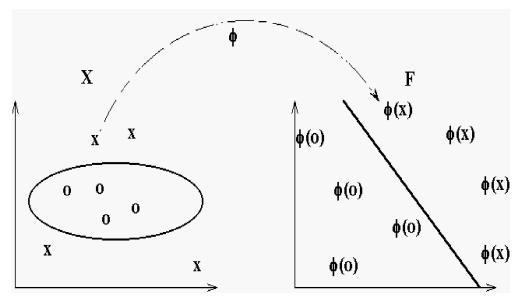Figure: Illustration of the mapping φ. On the left a set of samples in the input space, on the right the same samples in the feature space where the polynomial kernel K(x,y) (for some values of the parameters c and d) is the inner product. The hyperplane learned in feature space by an SVM is an ellipse in the input space.

Deep Learning: Instead of choosing a $\phi$ by hand, the strategy of DL is to learn $\phi$.

### Classification

Discriminate Function: Assigns sample point to a class.

• Binary Classification: $2$ classes
• Multi-class Classification: $K$ classes
• One-vs-all: train $K-1$ binary classifiers. However, you will see this leads to ambitious decision surfaces
• One-vs-one: train $K(K-1)/2$ combinations of binary classifiers for $K$. Still has ambitious dec. surfaces
• Multinomial: (this is the obvious choice I would code up before I even know the two other options existed). train 1 classifier w/ matrix of weights for all classes. The output is the dot product of the input $x$ w/ each set of weights for the respective class. So if you have 6 classes, you will get 6 scaler dot product values and the argmax corresponds to the winning class.
• What’s the difference with this and softmax?

#### Label Smoothing/Soft Targets

Keep in mind though that you might be limiting yourself to dogs and cat types in your training set: The model might not generalize as well to a Shiba Inu as the model that is only trained with two classes.

yep, by introducing more fine grain labels, you force the neural net to learn factors of variation

bonus points: if you use “soft targets”, youll get even better generalization as your neural net will learn to place different breeds near each other on the manifold

yep, think of it as smearing the probability mass across different dog breeds (instead of 1-hot encoding)

See, I always get a bump in accuracy when I use label smoothing… but I get a hit in macro averaged F1 and macro averaged PR AUC. (See knowledge distillation)

essentially take the 1-hot vector (0,0,1,0), and make the targets soft, or smooth out the labels (they are the same thing): (.1,.1,.9,.1).

The network learns a specific achievable value for each class, which prevents overfitting (also makes sure that in cross entropy the network also tries to decrease the score for the wrong labels, rather than just increase the score for the right labels)

generally you don’t make the smoothed labels sum to 1.

The inception paper (rethinking) introduced this idea.

### Logistic Regression

Where $\sigma$ is an activation function. You can also consider x as a basis function $\phi(x)$ as in Regressions.

The Logistic Regression(classification) coefficients are usually estimated using MLE. Unlike linear regression with normally distributed residuals, it is not possible to find a closed-form expression for the coefficient values that maximize the likelihood function, so that an iterative process must be used instead; for example Newton’s method or GD. This process begins with a tentative solution, revises it slightly to see if it can be improved, and repeats this revision until no more improvement is made, at which point the process is said to have converged.

#### Binary Cross Entropy Loss

Binary x-ent can be recovered by maximizing the likelihood of a binomial distribution.

### Regularization

Regularization is any process to help prevent overfitting in a model.

• Weight decay: L1, L2
• Bagging (Dropout)
• Early Stopping

#### Weight Decay

Ocam’s Razor: Among competing hypothesis, the simplest is the best. In parametric models, simplicity can be considered either:

• Less parameters: L1 Lasso
• Smaller parameters: L2 Ridge

L2 regularization assumes a prior belief that weights should be small and normally distributed around zero. Interesting!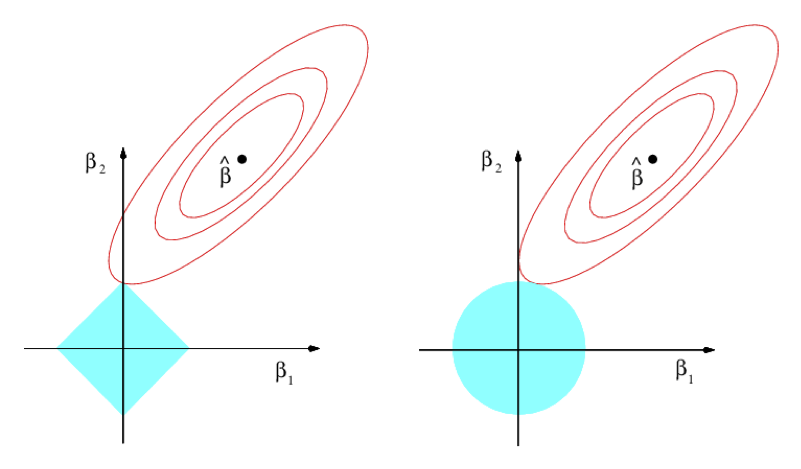This is an interesting visualization from ISL which shows a red unregularized convex loss function in two dimensions w/ parameters $\beta_1$ and $\beta_2$, where the optimal value is $\hat{\beta}$. It also shows the two constrained regions of the L1 and L2 norm, which are centered around zero and small in magnitude. Thus we can see the optimal regularized optimum is not $\hat{\beta}$ but rather the intersection of the two regions. Notice that this makes both losses have smaller magnitude and in the L1 case makes the solution space sparse as $\beta_1=0$!

So in other words, to summarize, the absolute value’s going to be a constraint region that has sharp corners. In high dimensions, you have edges and corners. And along an edge or a corner, if you hit there, you get a 0. So this is, geometrically, why you get sparsity in the Lasso.

Interesting L1 visualization: https://twitter.com/PierreAblin/status/1107625298936451073

### Ensembling

There is no universal model that preforms best on all data. Each model has tradeoffs, advantages/disadvantages against others (see no free lunch principle). In practice, it turns out that instead choosing the best model out of a set of models, if we combine many various, the results are better — often much better — and at little extra effort.

Creating such model ensembles is now standard. In the simplest technique, called

• bagging, we simply generate radom variates of the training set by revamping, learn an overfit classifier on each, and combine the results by voting. This works because it greatly reduces variance while only slightly increasing bias.
• boosting, training examples have weights, and thse are varied so that each new weak classifier can focus on the examples the previous ones tended to get wrong.
• stacking, the outputs of individual classifiers come the inputs of a “higher-level” larger that figure out how best to combine them.

### Tree Models (non parametric)

#### Decision Tree

Recursively Splits the decision space. Tries to partition the data in such a way to make PURE leafs – homogeneous groups.

• Good for non-linearly-seperable data, usually next choice after linear regression
• Prone to overfitting (high variance / low bias): Use bagging to alleviate: see random forrest
• Interpretable: visualize decision surface.
• lower-dimensional data is better / high dimensional will result in deep tree (read overfitted)
• Greedy Algorithm: not guaranteed to find the optimal solution.

Entropy

Purity/disorder measurement of set:
$H(x) = -\sum_{i=0}^n p_i\log_2p_i$
where $n$ is the class id.

##### Information Gain

Information Gain is the average entropy of the children subtracted from the the entropy of the parent :
$IG = H(parent) - (p) H(A) - (p-1) H(B)$
Where $p$ is the ratio of items in set A.

Algorithm

1. For the given branch (root if first iteration)
1. Find (exhaustively) feature/column w/ most potential information gain $f_i$
1. For each column, For each value, calculate info gain @value, and return largest.
2. Split the branch among the target classes with respect to $f_i$
3. For each split (2): Repeat Step #1, unless leaf!
##### Entropy Example

Where $p_i$ is the probability of class $i$. In the binary classification case, we can rollout the summation to:
$\text{Entropy}= -p\log_2p-q\log_sq$
Where p and q are the respective categories.

• What is the entropy of a homogenous set?
• $-1 \log_21 = 0$
• What is the entropy of a set w/ a 50/50 mix?
• $(-.5\log_2.5) - (.5\log_2.5) = 1$
X Y Class
Green Circle
Ping Cross
Green CircleFigure: 16 greens and 14 ping = 30 total

What is the potential entropy in the distribution above?
$\log_2(16/30) = -0.9 \\ \log_2(14/30) = -1.1 \\ \text{Entopy} = =(16/30)(-.9) - (14/30)(-1.1) = .99$
Very high entropy. This is a good column feature/column to split on.

##### Pruning

Basic D-trees massively overfit, so pruning helps simplify the tree in order to generalize better

#### Bagging

• Bootstrap Sampling: Random sample with replacement in order to lower variance (overfitting)
• RF: Averages the results of d-trees overfit on bootstrap samples.

The theory bagging, in a nutshell, is that you have multiple models that you blend together to reduce variance and make your predictions more stable. That’s how a random forest works, it is a combination of n_estimators decision tree models that use majority voting (in the case of Random Forest Classifier) or straight averaging (in the case of Random Forest Regressor). Random Forests are called Bagging Meta-Estimators. Bagging reduces variance by introducing randomness into selection of the best feature to use at a particular split. Bagging estimators work best with when you have very deep levels on the decision trees. The randomness prevents overfitting the model on the training data.

In bagging, we use many overfitted classifiers (low bias but high variance) and do a bootstrap to reduce the variance.

Bagging is short for Bootstrap Aggregation. It uses several versions of the same model trained on slightly different samples of the training data to reduce variance without any noticeable effect on bias. Bagging could be computationally intensive esp. in terms of memory.

The intuition behind bagging is that averaging a set of observations reduces the variance. Given $Z_n$ observations w/ variance $\sigma^2$, the variance of the mean $Z$ is given by $\frac{\sigma^2}{n}$. Hence a natural way to reduce the variance and hence increasing the predicting accuracy of a model is to take many samples of the training set and average the resulting predictions.

Examples: Random Forrest

#### Boosting

• Lowers bias while maintaining variance.

In boosting, we allow many weak classifiers (high bias with low variance) to learn form their mistakes sequentially with the aim that they can correct their high bias problem while maintaining the low-variance property.

Examples: AdaBoost, GradientBoost

##### AdaBoost

Boosting relies on training several (simple, usually decision stumps) models successively each trying to learn from the errors of the models preceding it. Boosting differes from other algorithms in that in addition to it gives weights to training examples (as opposed to linear models which apply weights on features). So in essence we can weight the scarcer observations more heavily than the more populous ones. Boosting decreases bias and hardly affects variance (unless you are very sloppy). Depending on your n_estimators paramenter you are adding another inner-loop to your training step, so the the price of AdaBoost is an exensive jump in computational time and memory.

### Kernels

Making data linearly separable by transforming it

https://www.quora.com/What-is-“kernel-methods”

### SVM

Maximum Margin Classifier. Tries to find 2 points, supports, which are the furthest away from the decision boundary. Trying to maximize the width of the street. Mathematical guarantee to find global maxima.

### Bias/Variance Tradeoff

If you model is overfitting, then it is high-variance: learning noise. If it is underfitting, it is too biased and not capturing the signal.

Often this is quantified by looking at the Learning Curves: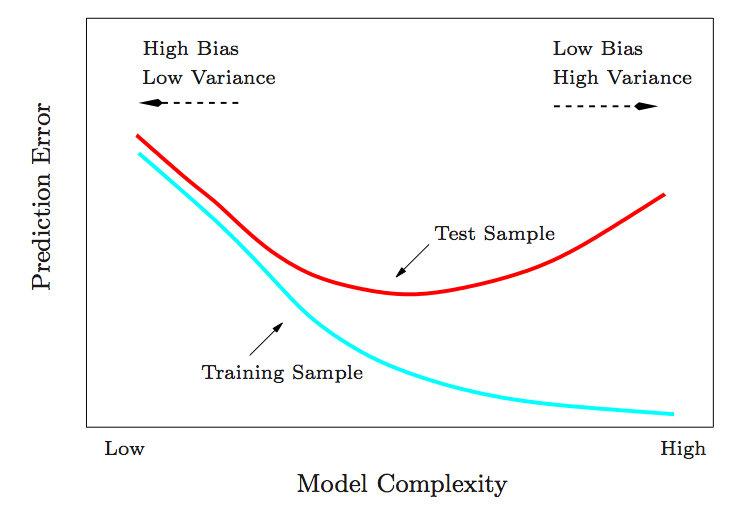How to remove variance (overfitting):

• Get more data
• Regularization
• Reduce (count/magnitude) of Parameters
• Bagging: Use less flexible model (eg linear)
• Early Stopping
• Also see data augmentation and batch norm*

How to remove bias (underfitting):

• Get more data

• More flexible model/parameters (eg Boosting)### Evaluation

• Precision: ability of the classifier not to label as positive a sample that is negative $\frac{TP}{ TP + FP}$
• Recall: ability of the classifier to find all the positive samples. (Min false negatives) $\frac{TP}{TP+FN}$
• Precision-Recall is a useful measure of success of prediction when the classes are very imbalanced.
• PR Curve: P&R at different thresholds cutoff thresholds in the binary classifier. The precision-recall curve shows the tradeoff between precision and recall for different threshold. A high area under the curve represents both high recall and high precision, where high precision relates to a low false positive rate, and high recall relates to a low false negative rate. High scores for both show that the classifier is returning accurate results (high precision), as well as returning a majority of all positive results (high recall). precision-recall curves are appropriate for imbalanced datasets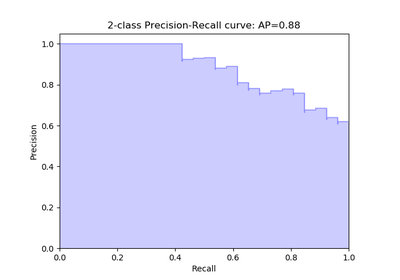• High Recall (Optimistic System): A system with high recall but low precision returns many results, but most of its predicted labels are incorrect when compared to the training labels
• High Precision (Pessimistic System): A system with high precision but low recall is just the opposite, returning very few results, but most of its predicted labels are correct when compared to the training labels.

#### ROC

ROC curves are appropriate when the observations are balanced between each classAUC

An evaluation metric that considers all possible classification thresholds.

The Area Under the ROC curve is the probability that a classifier will be more confident that a randomly chosen positive example is actually positive than that a randomly chosen negative example is positive

### Questions

• Linear Regression Assumptions:
• Linearity
• Constant variance of residual (stationarity)
• Normal distribution of variance with mean = 0
• What is stationary signal?:
• one which has a constant mean and variance
• Write out a function to calculate the AUC of an ROC curve
• The integral of the curve

## Optimization

### 0th Order Black Box

Only $f(x)$ can be evaluated

### 1st Order Gradient Based

$\nabla{f(x)}$ Can be evaluated

Vanilla GD has fixed shared learning rates across parameters/dimensions. Later innovations brought momentum. The latest innovations are in adaptive and individual learning rates across dimensions.

• Learning Rate: scales the gradient. Therefore the higher the learning rate, the more confidence you have in the quality/accuracy of your gradient. The higher the learning rate the faster you can possibly converge.
• Batch Size: The was an interesting analogy I heard about getting advice from a drunk person for directions. If he was very drunk you would only take little confidence in his directions, you’d go and ask the next person. However, if it was a sober person, you would have higher confidence in their direction and put more weight on it. The size of your batch is like how drunk the person is. A large batch is a sober person and small batch is a drunk person: $\abs{B}\propto \lambda$ . However, as your batches get smaller, the gradients have a lot more noise, which some may say will help not getting stuck in local minima.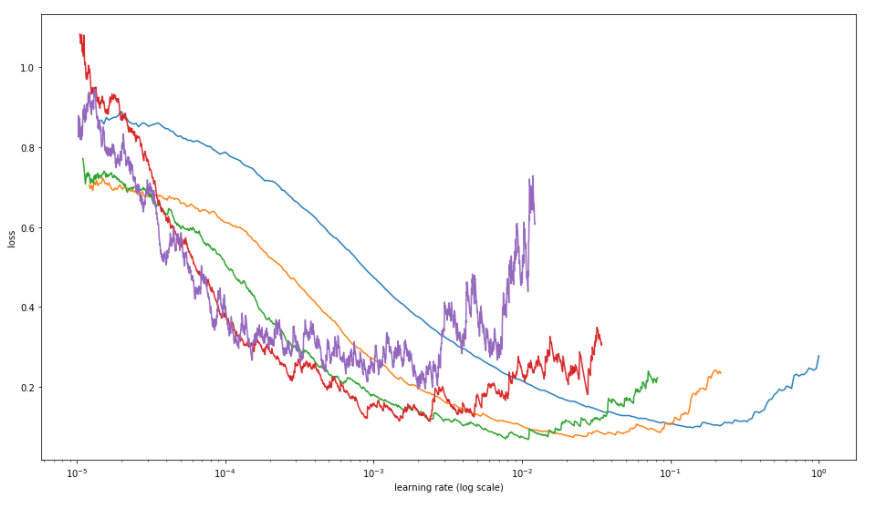Figure: chart from fastAI showing Loss correlation with LR and Batch size. Largest batch is blue which shows the smoothest curve and highest (optimal) learning rate. Purple is smallest batch size and you can see it has the lowest optimal learning rate. — the larger the batch the less noise in the gradient(loss)

#### Vanilla

(Vanilla) Gradient Descent is very sensitive to step size. In ill-conditioned setting, it’s very useful to have an adaptive step size: in regions of large variability of the gradient we would like to take small step sizes, while on regions with small variability we would like to take large step sizes.

#### Momentum

W/o Momentum W/ Momentum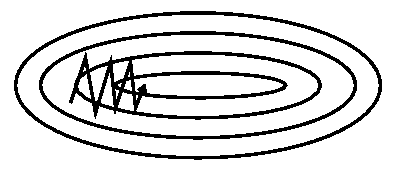Momentum  is a method that helps accelerate SGD in the relevant direction and dampens oscillations as can be seen in Image 3. It does this by adding a fraction $\gamma$ of the update vector of the past time step to the current update vector:
$v_t = \gamma v_{t-1} + \eta \nabla_\theta J( \theta) \\ \theta = \theta - v_t$
where $v_t$ can be seen as a exponentially decaying average of past gradients.

#### Adagrad

Adagrad is an adaptive learning rate method originally proposed by [Duchi et al]. Adapts the learning rate to the parameters, performing smaller updates (i.e. low learning rates) for parameters associated with frequently occurring features, and larger updates (i.e. high learning rates) for parameters associated with infrequent features. For this reason, it is well-suited for dealing with sparse data.

Independent parameter learning rates.

#Adagrad update
cache += dx**2 #vector initalized to 0 for every parameter
x += learning_rate * dx / (np.sqrt(cache) + 1e-7)


The cache continues to grow each step which helps to dampen the gradients. However, it will eventually become so large that it will dominate the denominator and cause learning to stop.

The fix for this is to decay the cache, so the system can keep learning: a la RMSProp.

#### Adadelta/RMSprop

Fixes the diminishing gradient problem w/ Adagrad.

In practice, deep nets Adagrad stops too early and RMSprop wins out.

#### Adam

RMSProp w/ Momentum

In addition to storing an exponentially decaying average of past squared gradients $v_t$ like Adadelta and RMSprop, Adam also keeps an exponentially decaying average of past gradients $m_t$, similar to momentum.

#### Learning Rate Decay

All of these methods have Learning Rate as a hyperparameter. This should always be decayed (step, exponentially)

### 2nd Order

$\nabla^2{f(x)}$ Can be evaluated

Kfac, Newton, Gauss-Newton, Quasi-Newton, (L)BFGS

#### Newton’s Method

Newton’s method can be used to find a minimum or maximum of a function $f(x)$: .
$x_{n+1} = x_n - \frac{f(x_n)}{f'(x_n)}$
The derivative is zero at a minimum or maximum, so local minima and maxima can be found by applying Newton’s method to the derivative. The iteration becomes:
$x_{n+1} = x_n - \frac{f'(x_n)}{f''(x_n)}$
LGBFS: batch mode only. Unlike mini-batch SGD, getting L-BFGS to work on mini-batches is more tricky and an active area of research.

## Neural Networks

Function Approximtors.

Multi-layer perceptron is a misnomer. It should be multi-layer logistic regression!

B/C of the non-linear activations, we have to solve the non-convex problem iteratively.

## Deep Learning

Layers of logistic regressions, separated by non-linearities. Each layer can learn a representation. Layer weights are learnt via back-propagation algorithm.

The non-linearities make it a composition of functions: $f(x)=f_3(f_2(f_1(x)))$ as opposed to linearities which would reduce to 1 function.

• Batch Norm: Normalization at inner layers (helps overfitting and convergence time – higher learning rates). When training Deep Neural Networks (DNNs), it is common practice to normalize or whiten features by shifting them to have zero mean and scaling them to have unit variance. It works by internally maintaining an exponential moving average of the batch-wise mean and variance of the data seen during training. The main effect of batch normalization is that it helps with gradient propagation—much like residual connections—and thus allows for deeper networks. Typically used after a convolutional or densely connected layer.
• How does Batch Normalization Help Optimization? (NeurIPS 2018): Argues that ICS (shift) has nothing to do w/ BN, but rather, it helps smooth the gradients and loss surface. https://twitter.com/aerinykim/status/1070188133810139136
• The NIPS loss surface paper form Washington that was on Arxive for a while shows how batch norm (and skip connections) smooth out the loss surface.
• Dropout: Regularization technique. Dropout is one of the most effective and most commonly used regularization techniques for neural networks, developed by Hinton and his students at the University of Toronto. Dropout, applied to a layer, consists of randomly “dropping out” (i.e. set to zero) a number of output features of the layer during training. The “dropout rate” is the fraction of the features that are being zeroed-out; it is usually set between 0.2 and 0.5. At test time, no units are dropped out, and instead the layer’s output values are scaled down by a factor equal to the dropout rate, so as to balance for the fact that more units are active than at training time.
• Ensembling Analogy: Dropout can been seen as an ensembling technique using bagging.
• Hinton on the inspiration for dropout: “I went to my bank. The tellers kept changing and I asked one of them why. He said he didn’t know but they got moved around a lot. I figured it must be because it would require cooperation between employees to successfully defraud the bank..
• CNNs: signal processing technique that is more parameter efficient than a FC.
• Activations: Introduce non-linearities, otherwise layers are pointless as can be combined into 1 linear operation
• Gradient Accumulation: If you cannot fit large batch sizes on your GPU, you can just average gradients over runs. Then apply them.

### Going Deep

In theory, the deeper you go the more expressive power you can achieve, however there are tradeoffs. Diminishing Gradients: Back-propagated errors become very small after a few layers, which makes learning ineffective.

• Skip-connections: The general idea is to reduce the distance between the signal coming from the networks loss and each individual layer. The way this is done is by adding a residual/direct connection between every layer and its predecessors. That way, the gradient can flow from each layer to its predecessors directly.
• This can be solved by pretraining, e.g. starting with initial weights that are close to the final solution.
• Dilated Convolutions

### CNNs

CNNs are motivated by 3 factors:

1. Locality: neighbor relationships in spatial data (images, audio, sequences) are ignored by FFNs (see: local receptive fields below)
2. Invariance: FFNs are not translation and scale invariant. They will memorize orientation and size. To mitigate this you would have to heavily regularize or do data augmentation of all possible combinations of orientation translations and size scales. (See subsampling below)
3. Parameter Scale: Fully Connected Networks don’t scale well especially to image data: e.g. [200 x 200 x 3] image, would lead to neurons that have 200x200x3 = 120,000 weights. Multiple layers of this would lead to overfitting due to too many parameters. A CNN layer on the same input volume would only need to learn 10x5x5x3 = 750 parameters! (Assuming 10 [5 x 5] filters over 3 channels ). (See Weight sharing below)

These limitations are remedied in CNNs by:

1. Local receptive fields
2. Weight sharing:
3. subsampling: Pooling

Parameters:

• Input volume size: $W$
• Receptive field size: $F$
• Stride: $S$
• Zero padding: $P$
• FIlter count: $K$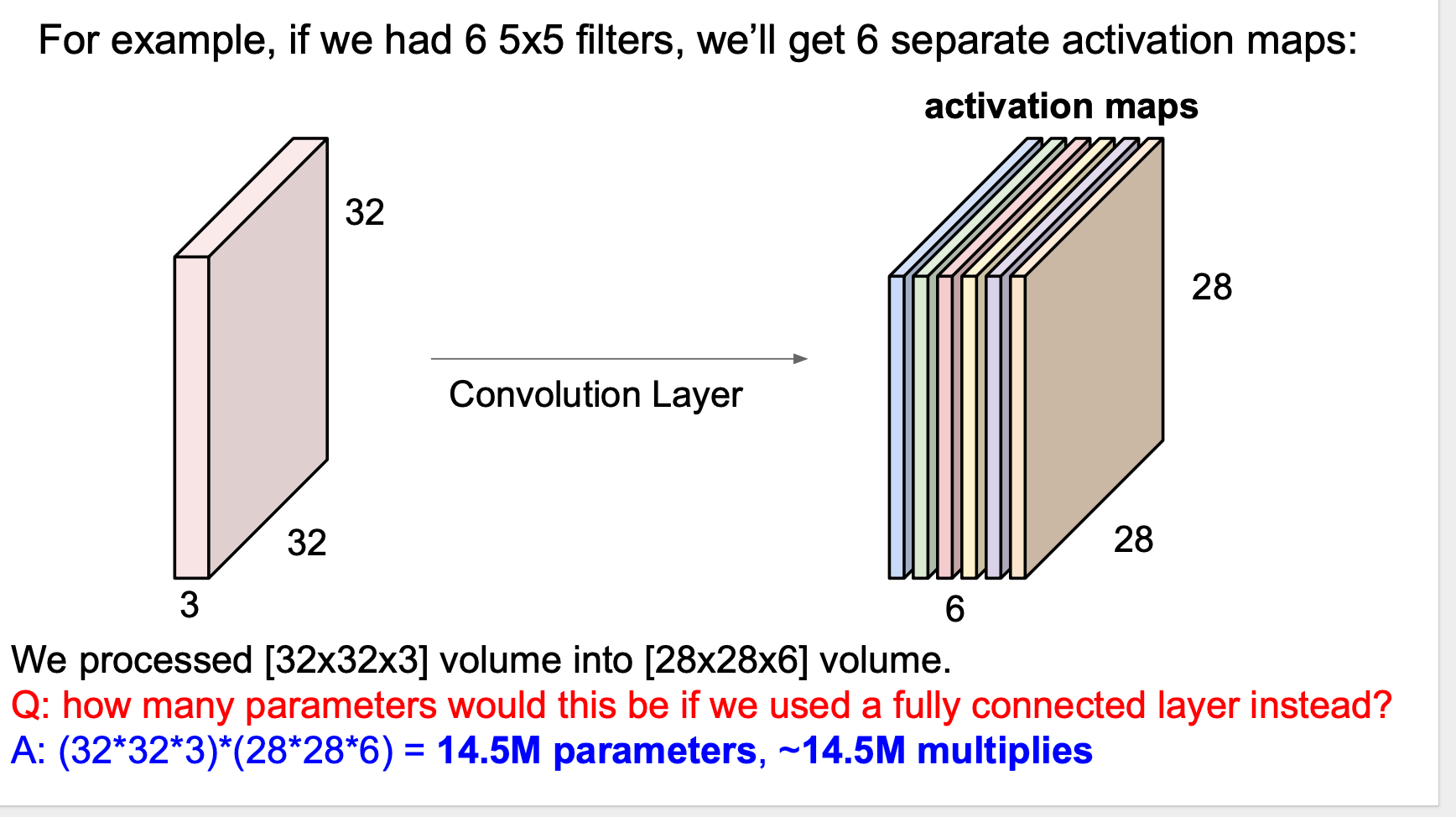The figure above is a great example of the advantage that weight sharing in CNNs give. 6 [5 x 5] filters on a [32 x 32 x 3] image give a give a [28 x 28 x 6] activation map with only 5 x 5 x 3 x 6 = 450 parameters. Compared to 14M parameters if we flattened the image and did it w/ a fully connected layer!

Parameters

• Number of Filters $f$
• Kernel Size of Filter $s$

Settings:

• Stride: how to move the window
• Padding:

Receptive Field: receptive field grows linearly w/ CNN layers. Dilated Convs let us grow receptive field exponentially w/ depth (more efficient in terms of params).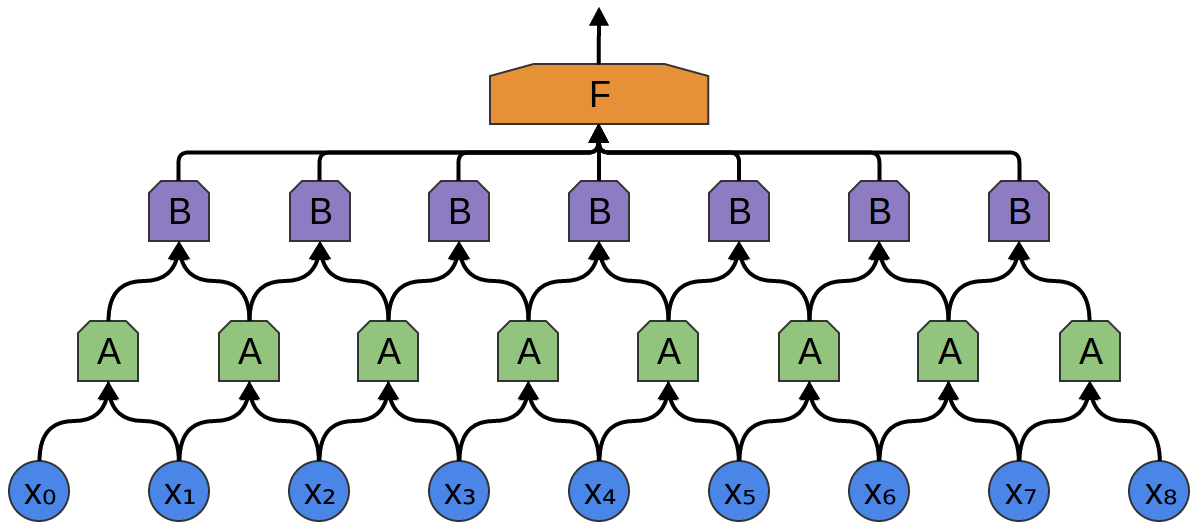Each “A” sees two inputs. Each “B” sees two “As” which have a common input so each “B” has a receptive field of 3. Each B is exposed to exactly 3 inputs. In other words, receptive field grows linearly w/ layers.

Such 1D convnets can be competitive with RNNs on certain sequence-processing
problems, usually at a considerably cheaper computational cost. Recently, 1D conv nets, typically used with dilated kernels, have been used with great success for audio
generation and machine translation. In addition to these specific successes, it has long
been known that small 1D conv nets can offer a fast alternative to RNNs for simple tasks
such as text classification and timeseries forecasting.

#### Causal

Like LSTMs, causal convolutions can model sequences with long-term dependencies. This is achieved in two ways: stacking convolutional layers (with padding, every convolutional layer preserves the shape of the input), and dilation: insertion of gaps into the convolutional filters (otherwise known as atrous convolutions). convolutional filters applied to the sequence in a left-to-right fashion, emitting a representation at each step. They are causal in that the their output at time t is conditional on input up to t-1: this is necessary to ensure that they do not have access to the elements of the sequence we are trying to predict

Spotlight CNN Sequential model: https://maciejkula.github.io/spotlight/sequence/representations.html#spotlight.sequence.representations.CNNNet

The convolution is causal because future states are never part of the convolution’s receptive field; this is achieved by left-padding the sequence.

Dilation: setting this to a number greater than 1 inserts gaps into the convolutional layers, increasing their receptive field without increasing the number of parameters. Stacked dilated convolutions enable networks to have very large receptive fields with just a few layers, while preserving the input resolution throughout the network as well as computational efficiency.

Dilated convolutions AKA atrous convolutions AKA convolutions with holes are another method of increasing the receptive field without angering the gradient gods. When we looked at stacking layers so far, we saw that the receptive field grows linearly with depth. Dilated convolutions let us grow the receptive field exponentially with depth.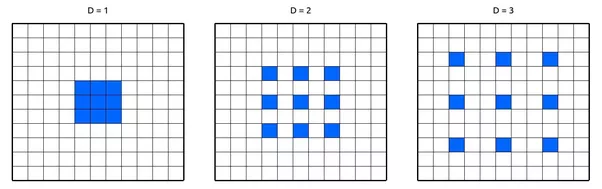Here we have a 3X3 filter applied with a dilation of 1,2 and 3. With a dilation of 1 we have a standard convolution. With a dilation of 2 we apply the same 3X3 filter but use every second pixel

Oord, Aaron van den, et al. “Wavenet: A generative model for raw audio.” arXiv preprint arXiv:1609.03499 (2016).

#### Separable

P262 of the Keras book

#### Capsules

CNNs are translation invariant, however, we want them go equivariant!

One of the goals of subsampling/pooling is to introduce invariance to the model. Meaning it tries to make the neural activities invariant to small viewpoint changes.

Input > Conv > relu > pooling

Subsampling or pooling in convents looses the spatial relationships in the images. for example a face isn’t an arbitrary combination of eyes + nose + ears + mouth, it’s a specific arrangement of the pieces.

Pooling throws away information about the precise position of the entity within the region. Pooling reports the activity of the most active neurons and then forgets where they are, which gives you a small amount of translational variance.

Capsules are also robust against adversarial attacks b/c they learn the distribution of each class. This can be used to check for adversarial input from a different distribution.

Image Classification w/ Capsules

1 capsule/class in the top layer. so most examples only have 10 capsules (eg mnist) in first layer, but ImageNet would have 1000. it is at least O(n) where n is the number of classes. routing by aggreement causes it to be slow to train b/c of the inner loop.

### RNNs

Stateful layers:

• RNN
• GRU
• LSTM

https://medium.com/@TalPerry/convolutional-methods-for-text-d5260fd5675f

Feed characters into layers of RNNs and get final state at end that represents the sequence.

LSTMs (vanishing gradients)

With a basic RNN cell, we see a massive drop in performance when it comes to long sequences and the network needs to remember patterns which have occurred way at the beginning to infer things correctly at a current time step. And this is because of exploding and vanishing gradients.

LSTMs, which can remember information from the way past and also selectively forget stuff that is not required.

### Residual/Skip Connections

“How do I make my network very deep without losing the gradient signal?”

The general idea is to reduce the distance between the signal coming from the networks loss and each individual layer. The way this is done is by adding a residual/direct connection between every layer and its predecessors. That way, the gradient can flow from each layer to its predecessors directly.

Dilated Causal Convs can possibly replace LSTMs

Causal Convs: layers of Convs that don’t look ahead.

## Transfer Learning

Learning in one domain and transferring it to another.

## Domain Transfer/Adaptation

One application of this is learning from a simulation .

## Applied ML

### Recommender Systems

Representation Learning. Most popular Rec Sys these days can be considered Representation Learning approaches. They all learn some dense representation of items and then uses that data structure to make predictions. This has a nice auxiliary product of similarity tables, however, it is also not directly modeling the recommendation task.

• Representation Learning
• Item-Item (Lindon. AMZN). Learns a symmetric item-item similarity table.
• MF: Latent Factor Models. Learns User and Item embeddings. No concept of time.
• ALS MF
• Pairwise $P(i > j \given U)$ Ranking objectives
• BPR
• Next Item Models: $P(i_{t+1} \given U, C)$. Better objective more aligned w/ most rec tasks. Weak concept of time.

Cold Users

Next item models, or models where a user representation isn’t just an ID are more robust to the cold-user problem.

Cold Items

Most (all) LF architectures are vulnerable to this and the only real and popular way to deal with it is content filtering or hand made features.

####

### IR

• Two-Phase System: First stage is candidate generation from full corpus: high precision/fast response. The second stage is a high recall ranker which is more complex and uses more data sources.
• Personalized Search: The First stage can be a recommender system or a search engine which is personalized. The second stage can also use personalization give a user and context.

#### LTR

supervised task to rerank a list for optimal order. Data can be relevance judgements or log data. Log data gives you implicit ranking preference from clickstreams. With clickstream data you can either model clicks or ranking.

Architectures:

• RankNet: siamese network w/ AUC objective $P(i>j)$
• LambdaRank: directly optimizes NDCG objective
• YouTube: models user video watch time as ranker (not LTR per se)

#### Ranking Metrics

##### DCG

As you can see the denominator discounts the relevance more and more as the rank goes down.

### Unbiased Bandit Data

Approaches that use log data, such as RecSys and LTR have to deal w/ a bias issues. This data, also called Bandit Data, is generated by a system. Compare this to the classical supervised learning approach w/ ground-truth labels, log data is biased by the system from which it is made.

### Object2Vec

Distributed Representation/Representation Learning are popular across all fields currently. It all started w/ word2vec where items are clustered in an embedding space with respect to how similar they are in some $\text{context}$. These dense representations are then commonly used for downstream tasks like features for classification.

• Uber learns sentence embeddings using doc2vec where a sentence is from a chat message. Not sure what is the context here. And how to use it of unobserved sentences….

#### Word2Vec

Given a context, surrounding words, predict the target word. Error propagates back down to the embeddings.

• CBow: given context, predict target $P( T \given C)$
• Skip-gram: given target, predict context $P(C\given T)$
• Glove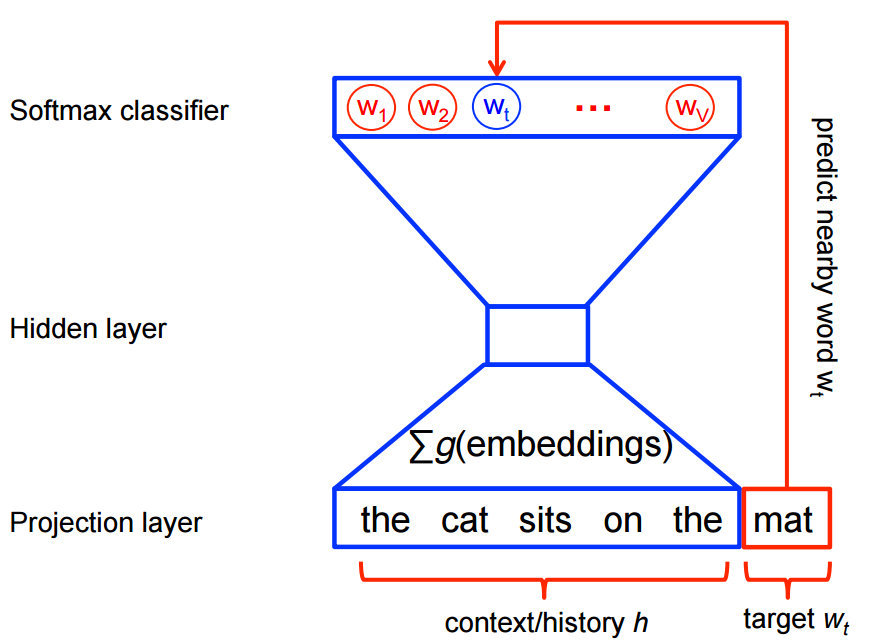Figure: Skip-gram architecture.

The figure above w/ the context “the cat sits on the” and the target “mat” can be generalized into many general use cases. For example Uber Eats learns embeddings where the context is the search query and the target is the item the user converted. For example: “Dan Dan Noodles” and {Item A}

##### Candidate Sampling

Softmax over 5000 classes is slow.
$S(x_i) = \frac{e^{f(x_i)}}{\sum_j e^{f(x_j)}}$
So if you have 5000 classes to evaluate the softmax layer you need to do 5000 forward passes through your function $f$ fro the different values of $x_i$. Computational Complexity: $O(\abs{V})$

This has motivated work into approximate softmax methods often called Candidate Sampling:

• Hierarchical Softmax
• Sampled Softmax
• Noice Contrastive Estimate (NCE)

### Text Classification

Given a sentence model the probability of a it’s label $P(y\given X)$ where x is a variable length list of words.

Nice guide here: https://developers.google.com/machine-learning/guides/text-classification/

Because bag-of-words isn’t an order-preserving tokenization method (the tokens gen-
erated are understood as a set, not a sequence, and the general structure of the sen-
tences is lost), it tends to be used in shallow language-processing models rather than
in deep-learning models. Extracting n-grams is a form of feature engineering, and
deep learning does away with this kind of rigid, brittle approach, replacing it with hier-
archical feature learning.

##### BOW

Classic approach which encodes the sentences/observations into fixed length feature vector length $\abs{V}$ . The encoding can be simple indicators for a word or counts or more popularly tf-idf counts.

Once you have your fixed length feature, you can use any type of classifier.

##### Sequential

Instead of a fixed length feature vector w/ frequency information, we learn/use an embedding for each word/token.

• N-Gram: groupings of sequential words of Len N
• BOW: Fixed length feature vector of N-gram counts over $V$.
• Independence between BOW features is assumed, however, that is mitigated a bit by the fact the BOW features may infect be N-Grams which will encode some dependence (sequence info)
• Feed-Forward:
• Ngrams: fixed length feature vector of ngrams over $V$ (very high dimensional)
• BOW: fixed length feature vector of averaged ngram embeddings (low dimensional)
• RNN: feed embeddings to RNN layers
• CNN: Convolve over ngram sequence of embeddings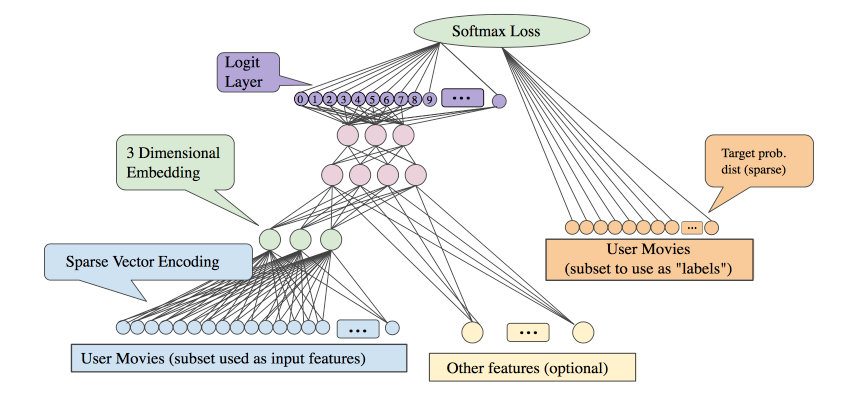Figure: A sequential Feed-forward MLP(DNN). Note for the 3D embedding layer: Although the diagram is ambiguous, the embeddings for each activated movie are either added or averaged.

One strategy to combine the speed and lightness of convnets with the order-sensitivity of RNNs is to use a 1D convnet as a preprocessing step before an RNN (see figure 6.30). This is especially beneficial when you’re dealing with sequences that are so long they can’t realistically be processed with RNNs, such as sequences with thousands of steps. The convnet will turn the long input sequence into much shorter (downsampled) sequences of higher-level features. This sequence of extracted features then becomes the input to the RNN part of the network.

NLP

• Named Entity Recognition
• Coreference
• Part of Speech Tagging

## Anomaly Detection

Isolation Forests: Unsupervised. Overfit a a bunch of trees (forest) and then the average path length to a point is called the anomaly score. Put in a threshold and you have a classifier. The random partitioning, on average, creates shorter paths for anomalies/outliers, which is intuitive b/c it should be hard to separate inliers.

Permalink: machine-learning-glossary

Tags:

Last edited by Alex Egg, 2019-06-20 12:16:56